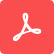# Lecture 1: A Visual Introduction to Turbulence and its Prediction in CFD

Dr. Spalart will discuss the intricacies of turbulent flows and why we should use turbulence models as approximations instead of fully resolving the flow physics.

Share On:CFD Essentials - Lecture 1

Hello. I'm Philippe Spalart. After a career at NASA and Boeing, I'm in charge of turbulence at Flexcompute. Today, I want to get you started in turbulence without equations and give you a feeling of how we're going to address it in CFD.

So first I'll show you a direct numerical simulation of a boundary layer. It’s the work of Strelets funded by Boeing. It's a solution of the Naviers -Stokes equations with billions of grid points. The fluid is coming from the left. We have the boundary layer that was made turbulent. You see what we call eddies, vortices, structures, vortex loops and all that that are tumbling. You notice right away that the eddies are much smaller near the wall than in the outer part of the boundary layer. This flow has a shockwave here and the pressure rise of the shockwave is causing it to separate. You can see how the fluid with the fine structures is coming off the wall and then slowly mixing with the rest of the turbulent fluid. You notice a pretty sharp interface between turbulent fluid and non-turbulent fluid. So this shows that we can simulate turbulence directly, but this is for a postage stamp size area for a millisecond. We need to deal with that fact.

So let's look at length scales. The boundary layer thickness is well under a centimeter at the leading edge, the structures near the wall are the order of a few micrometers and the timescales are a few microseconds. Of course we need to predict the lift and the drag of the entire airplane. So the scale there is of the order of 100 meters. We can see that the ratio of scales is huge. If we did a brute force approach to gridding, we'd be looking at 1024 grid points. The best we can do today is around 1010. So you can see that there are so many orders of magnitude differences that actually it may be that we never can build a computer large enough to do this. Gpu or not. Quantum computing or not. So we're going to have to do some modeling.

Another thing about the nature of turbulence is the energy cascade. There's an excellent simulation by Beck and Gassner. So this is a periodic geometry. Think of Lego blocks lined up in all three directions, repeating forever. We're plotting vorticity, which is how fast is the fluid spinning? This is the initial condition which in the Taylor-Green vortex is very simple, just sines and cosines. But once you let the Navier-Stokes equations proceed the spinning regions start twisting around each other, stretching and becoming smaller. If you keep going, then you get to a state that looks like this, where the energy is now spread over all the length scales. Now you can still see that periodic cube. What's interesting here is that there are some regions that have not yet filled with turbulence. But the ratio of the size of the box to the size of the smallest eddies is a function of the Reynolds number of the simulation which is controlled by the viscosity. So I call this turning potatoes into spaghetti. In 1941 in Moscow, Kolmogorov had a simple theory, predicting the famous K -5/3 spectrum. Everybody looks for that in their simulations. But again, the Reynolds number here is 1600 compared with roughly 10 million for the airplane boundary layer and probably even more for a submarine.

So now, Reynolds averaging of a turbulent flow field. Again, this simulation is by Strelets. On the left we have a large eddy simulation, which is similar to the direct simulation, but doesn't resolve the smallest eddies. But the flow is again three dimensional and unsteady. What we see here is the yellow counterclockwise vortex coming off, then it's going to propagate to the right. it will then have an opposite vortex coming from the upper surface. So it's a very complex flow. The Reynolds averaging process consists of, if we pick a point in space and time average it or averaging along a third direction, what comes out is this object. We're again plotting the vorticity, but it's now smooth and symmetric. The streamlines make it look like the flow is just spinning. So you can see that coming from this rich, very three dimensional and time dependent situation, we have considerably stripped down the information. The principle of turbulence modeling is to find equations that will give you this averaged field to a fairly good approximation. It's not easy. Now if you ask me, why not do the large eddy simulation? Well, if this is the body of a helicopter or the smokestack of a factory, you could spend the millions of grid points. But this could be the spoke on the wheel of your bicycle; then you don't want to spend a million grid points per millimeter of spoke length. So then we would do averaging and there we need to get a good enough drag for the spokes. Thank you.